Win up to 100% scholarship on Aakash BYJU'S JEE/NEET courses with ABNAT Win up to 100% scholarship on Aakash BYJU'S JEE/NEET courses with ABNAT

# JEE Main 2021 July 20 Shift 1 Maths Question Paper

JEE Main 2021 July 20 shift 1 Mathematics question paper and solutions are given here. JEE aspirants and students likewise can quickly access the question paper as well as the PDF from this page. The solutions to each of the questions have been prepared by our specialized team of experts. The solutions are structured in a step by step format which will ultimately help each student preparing for the exam to easily understand the problems. They will find only the correct answers to the questions asked in the JEE Main 2021 Mathematics paper.

Going forward, students can use this question paper and solutions as a reference to practise solving different types of problems expertly as well as analyze their performance. In the end, students will develop better problem-solving speed, analysis capability and finally score higher marks in the upcoming exams.

July 20 Shift 1 – Maths Question Paper and Solutions

Question 1: The mean of 6 numbers is 6.5 and its variance is 10.25. If 4 numbers are 2,4,5 and 7, then find the other two.

Answer: a = 10 and b = 11 or a = 11 and b = 10

Let a and b be the other 2 numbers.

$$\begin{array}{l}\bar{x}=6.5\end{array}$$

So (a + b + 2 + 4 + 5 + 7)/6 = 6.5

⇒ (a + b + 18)/6 = 6.5

⇒ a + b = 21 …(i)

σ2 = 10.25

⇒ (a2 + b2 + 22 + 42 + 52 + 72)/6 – 6.52 = 10.25

⇒ (a2 + b2 + 4 + 16 + 25 + 49)/6 – 42.25 = 10.25

⇒ a2 + b2 = 221…(ii)

Solving (i) and (ii)

We get a = 10 and b = 11 or a = 11 and b = 10.

Question 2: Find the coefficient of x256 in (1- x)101(x2 + x + 1)100

(1- x)101(x2 + x + 1)100 = (1 – x)(1- x)100(x2 + x + 1)100

= (1 – x)(1 – x)100(x2 + x + 1)100

= (1 – x)(1 – x3)100

= (1 – x3)100 – x(1-x3)100

First term will not have x256.

Coefficient of x256 is -1×coefficient of x255 in (1-x3)100

= -1(- 100C85)

= 100C85

Question 3: If

$$\begin{array}{l}\vec{a}\end{array}$$
,
$$\begin{array}{l}\vec{b}\end{array}$$
,
$$\begin{array}{l}\vec{c}\end{array}$$
are three mutually perpendicular vectors equally inclined to
$$\begin{array}{l}\vec{a}+\vec{b}+\vec{c}\end{array}$$
at angle θ, then find the value of 36 cos2 2θ.

$$\begin{array}{l}\vec{a}.\vec{b} = \vec{b}.\vec{c}=\vec{c}.\vec{a}=0\end{array}$$
$$\begin{array}{l}(\vec{a}+\vec{b} +\vec{c}). (\vec{a}+\vec{b} +\vec{c})= \left | \vec{a} +\vec{b}+\vec{c}\right |^{2}\end{array}$$
$$\begin{array}{l}\left | \vec{a} \right |^{2}+\left | \vec{b} \right |^{2}+\left | \vec{c} \right |^{2}= \left | \vec{a} +\vec{b}+\vec{c}\right |^{2}\end{array}$$
…(i)

$$\begin{array}{l}\vec{a}.(\vec{a} +\vec{b}+\vec{c})=\left | \vec{a} \right |\left | \vec{a}+\vec{b} +\vec{c}\right |\cos \theta\end{array}$$
$$\begin{array}{l}\left | \vec{a} \right |^{2}=\left | \vec{a} \right |\left | \vec{a}+\vec{b} +\vec{c}\right |\cos \theta\end{array}$$
$$\begin{array}{l}\left | \vec{a} \right |=\left | \vec{a}+\vec{b} +\vec{c}\right |\cos \theta\end{array}$$

Similarly

$$\begin{array}{l}\left | \vec{b} \right |=\left | \vec{a}+\vec{b} +\vec{c}\right |\cos \theta\end{array}$$
$$\begin{array}{l}\left | \vec{c} \right |=\left | \vec{a}+\vec{b} +\vec{c}\right |\cos \theta\end{array}$$

$$\begin{array}{l}\left | \vec{a} \right |=\left | \vec{b} \right |=\left | \vec{c} \right |\end{array}$$

From (i) we get

$$\begin{array}{l}3\left | \vec{a} \right |^{2}=\left | \vec{a} + \vec{b} + \vec{c} \right |^{2}\end{array}$$
$$\begin{array}{l}3\left | \vec{a} +\vec{b}+\vec{c}\right |^{2}\cos ^{2}\theta =\left | \vec{a} + \vec{b} + \vec{c} \right |^{2}\end{array}$$

⇒ cos2θ = 1/3

1 + cos 2θ = 2cos2θ

36 cos2 2θ = 36(2×1/3 – 1)2

= 36/9

= 4

Question 4: If f(x) =

$$\begin{array}{l}\left\{\begin{matrix} \sin x-e^{x} ,&x<0 \\ a+[-x], &0\leq x< 1 \\ 2x-b, & x\geq 1 \end{matrix}\right.\end{array}$$
is continuous in (-∞,1], find the value of a+b.

Answer: a + b = 3

f(x) =

$$\begin{array}{l}\left\{\begin{matrix} \sin x-e^{x} ,&x<0 \\ a+[-x], &0\leq x< 1 \\ 2x-b, & x\geq 1 \end{matrix}\right.\end{array}$$

= -2

f(1) = a + (-1) = a – 1

f(1+) = 2 – b

f(1) = f(1+)

⇒ a – 1 = 2 – b

⇒ a + b = 3

Question 5: The number of solutions of tan-1√(x(x+1)) + sin-1√(x2+x+1) = π/2 is

Given tan-1√(x(x+1)) + sin-1√(x2+x+1) = π/2

⇒ tan-1 √(x(x+1)) = π/2 – sin-1√(x2+x+1)

⇒ tan-1 √(x(x+1)) = cos-1√(x2+x+1)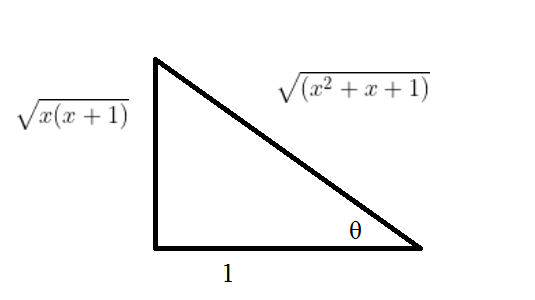⇒ cos-1 1/√(x2+x+1) = cos-1√(x2+x+1)

⇒ 1/√(x2+x+1) = √(x2+x+1)

⇒ (x2+x+1) = 1

⇒ x2 + x = 0

⇒ x(x+1) =0

⇒ x = 0 or x = -1

Therefore, the number of solutions is 2.

Question 6: There are 6 batsmen, 7 bowlers and 2 wickets keepers. Find the number of ways of forming a team of 11 players having at least 4 batsmen, at least 5 bowlers and at least 1 wicket keeper.

a. 567
b. 525
c. 462
d. 777

Team Size =11 players

Case 1: 4 batsmen and 5 bowlers and 2 wicket-keepers

6C4 × 7C5 × 2C2

= (6×5/2) × (7×6/2) × 1

= 315

Case 2: 4 batsmen and 6 bowlers and 1 wickets keeper

6C4 × 7C6 × 2C1

= (6×5/2) ×7×2

= 210

Case 3: 5 batsmen and 5 bowlers and 1 wickets keeper

6C5 × 7C5 × 2C1

= 6×(7×6/2) ×2

= 252

So total number of ways = 315 + 210 + 252

= 777

Hence option d is the answer.

Question 7: All the letters of the word EXAMINATION are arranged. Find the probability that ‘M’ is at the 4th position.

a. 2/11
b. 1/11
c. 4/11
d. 8/11

EXAMINATION

Letters: AAIINNE T O X M

Total possible words

n(S) = 11!/2!3

Now when M is at 4th position

n(E)=10!/(2!)3

P (M is at 4th position) = (n(E))/(n(S))

= 1/11

Hence option b is the answer.

Question 8: Find the number of integral terms in the expansion of

$$\begin{array}{l}(4^{\frac{1}{4}} + 5^{\frac{1}{6}})^{120}\end{array}$$

a. 1
b. 21
c. 20
d. 11

T(r+1) = 120Cr 4(120 – r)/45r/6

= 120Cr 2(120 – r)/25r/6

For integral terms, r should be even and multiple of 6

i.e., r should be a multiple of 6.

r can be 0,6, 12,…,120

Therefore, the total number of integral terms = 21

Hence option b is the answer.

Question 9: If

$$\begin{array}{l}\lim_{x\to0}\left \{ 2-\cos x\sqrt{\cos 2x} \right \}^{\frac{x+2}{x^{2}}}=e^{\alpha }\end{array}$$
, then α = ?

$$\begin{array}{l}\lim_{x\to0}\left \{ 2-\cos x\sqrt{\cos 2x} \right \}^{\frac{x+2}{x^{2}}}=e^{\alpha }\end{array}$$
(1 form)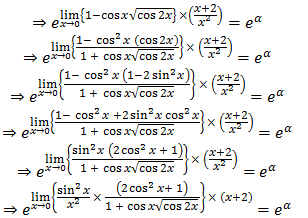⇒ e(2+1)2/(1+1)

= eα

⇒ e3 = eα

= α = 3

Question 10: Evaluate ∫-11 log (√(1 – x) + √(1 + x))dx

Answer: 2 ln(2) – (1 – π/2)

f(-x) = f(x)

⇒ f is even

∴ I = 2∫01 log ((1 – x) + √(1 + x))dx…(i)

We will solve it by integration by parts.

Let u = ln⁡(√(1-x)+√(1+x)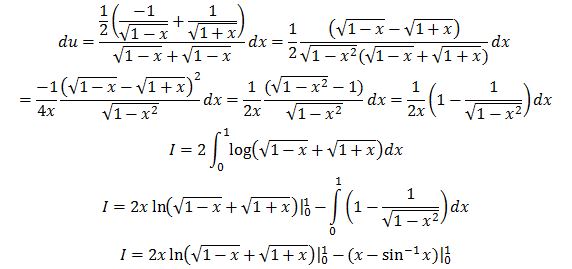I = 2 ln(2) – (1 – π/2)

Question 11: The logical equivalence of the Boolean expression ~(p∧~q)∨(q∨~p) is

~(p∧~q) ∨ (q∨~p)

≡ (~p∨q) ∨ (q∨~p)

≡ ~p∨q

≡ p → q

Question 12: Consider the curve y2 = 2x and point A(2, 2). If the normal at A intersects the curve again at point B and the tangent at A intersects the x-axis at C, then area of ∆ABC is

Equation of tangent at A

yy1 = x+x1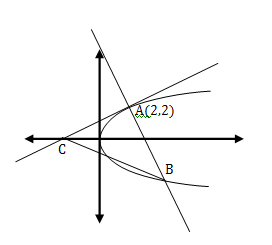⇒2y = x+2

Tangent at A intersects the x-axis at C

C(-2, 0)

Equation of normal at A

y+ 2x = 6

Solving normal equation with y2 = 2x, we get B(9/2,-3)

So, area will be ½ × AC×AB

= ½ ×2√5×√[(25/4) + 25]

= 25/2

Question 13: If for vectors A and B, A.B = |A×B|, then |A-B| is

a. √(A2 + B2√(2AB))
b. √(A2 + B2 – √(2AB))
c. √(A2 + B2 – √2 AB)
d. √(A2 + B2 + √2 AB)

A.B = |A×B|

⇒ cos θ = sin θ

⇒ tan θ = 1

⇒ θ = π/4

|A-B|2 = A2 + B2 – 2A.B

= A2 + B2 – 2AB cos π/4

= A2 + B2 – √2AB

⇒ |A – B| = √(A2+ B2 – √2 AB)

Hence option c is the answer.

Question 14: If the roots of the quadratic equation x2 + 31/4x + 31/2 = 0 are α and β, then the value of α9612 – 1) + β9612 −1)

a. 50⋅324
b. 51⋅324
c. 52⋅324
d. 104⋅324

x2 + 31/2 = – 31/4x

⇒ x4 + 3 = -31/2x2

⇒ x8 + 9 + 6x4 = 3x4

⇒ x8 + 9 + 3x4 = 0

α8 = -9 – 3α4

And α12 = -9α4 – 3α8

= -9α4 -3(-9-3α4)

= 27

Similarly, β12 = 27

α9612 – 1) + β9612 – 1) = 278.26 + 278.26

= 52.324

Hence option c is the answer.

Question 15: In ∆ABC, if AB = 5, ∠B=cos-1 (3/5) and the radius of circumcircle of triangle is 5. Then the area of ∆ABC is

a. 6+8√3
b. 3+4√3
c. 3+8√3
d. 6+4√3

cos ⁡B = ⅗

⇒ sin⁡B = 4/5, R = 5

b = 2R sin⁡B = 8

AB = c = 5

By cosine rule,

cos ⁡B = (a2+c2-b2)/2ac = 3/5

⇒ (a2+25-64)/(2a(5))

= 3/5

⇒ a2-39 = 6a

⇒ a2-6a-39 = 0

⇒ a = ((6+8√3))/2

⇒ a = 3+4√3

∆ = abc/4R

= 6+8√3

Hence option a is the answer.

Question 16: If the shortest distance between the lines

$$\begin{array}{l}r_{1}=\alpha\hat{i}+2\hat{j}+2\hat{k}+\lambda (\hat{i}-2\hat{j}+2\hat{k})\end{array}$$
, λ∈R, α>0 and
$$\begin{array}{l}r_{2}=-4\hat{i}-\hat{k}+\mu (3\hat{i}-2\hat{j}-2\hat{k})\end{array}$$
, µ∈R is 9, then the value of α is

a. 2
b. 4
c. 6
d. √6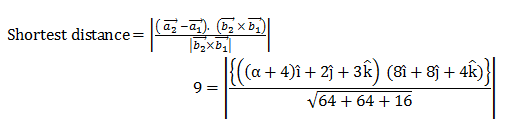⇒ |(α + 4) ×8 + 16 + 12| = 108

⇒ (α + 4) ×8 = 80 (since α >0)

⇒ α = 6

Hence option c is the answer.

Question 17: Let y = mx+c, m>0 be the focal chord of y2 = −64x which is tangent to (x+10)2+ y2 = 4. Then the value of 4√2(m+c) is equal to

Focus of parabola is (−16,0)

So, −16m + c=0

⇒c = 16m …(i)

Now slope form of tangent to the circle is given by

y = m(x+10) ± 2√(1+m2)

So c = 10m ± 2√(1+m2) …(ii)

So, from (i) and (ii)

16m = 10m ± 2√(1+m2)

⇒9m2 = 1 + m2

⇒ m = 1/2√2

⇒ c = 16m

= 8/2√2

4√2 (m+c) = 34

Question 18: A continuous differentiable function f(x) is increasing in (-∞, 3/2) and decreasing in (3/2, ∞). Then x = 3/2 is

a. point of local maxima
b. point of local minima
c. point of inflection
d. None of these

A rough graph of f(x) can be drawn as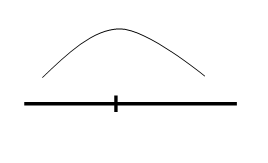Thus x = 3/2 is a point of local maxima.

Hence, option a is the answer.

Question 19: If z and ω are complex numbers such that |zω| = 1, arg⁡(z) – arg⁡(ω) = (3π )/2 then find arg

$$\begin{array}{l}\left ( \frac{1-2\bar{z}\omega }{1+3\bar{z}\omega } \right )\end{array}$$

a. π/4
b. -π/4
c. 3π/4
d. -3π/4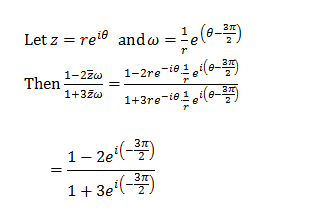= (1-2i)/(1+3i)

= -½ – ½ i

arg( -½ – ½ i) = -3π/4

Hence option d is the answer.

Question 20: If an invertible function f(x) is defined as f(x) = 3x – 2, g(x) is also an invertible function such that f-1 (g-1 (x) )= x – 2, then g(x) is

a. (x-8)/3
b. (x+8)/3
c. (x-3)/8
d. (x+3)/8

f-1 (g-1(x) ) = x-2

⇒ f(x-2) = g-1 (x)

3(x – 2) – 2 = g-1(x)

3x – 8 = g-1 (x)

g-1 (x) = 3x – 8

or x = 3g(x) – 8

So g(x)=(x + 8)/3

Hence option b is the answer.

Question 21: The probability of selecting integers a ∈ [-5,30], such that x2 + 2(a+4)x – 5a + 64 >0 for all x∈ R is:

x2+ 2(a+4)x – (5a – 64)>0

D<0

Therefore 4(a+4)2 + 4(5a – 64) < 0

⇒ a2 + 13a – 48<0

⇒ (a + 16)(a – 3)<0

So, a ∈ (-16,3)

Total integers are 18

Probability = 18/36 = 1/2

Question 22: If ∫0a ex-[x] dx = 10e – 9, then the value of a is (where [.] is greatest integer function)

a. 9 + ln⁡ 2
b. 10 + ln⁡ 2
c. 10
d. 9

Let a = K + λ, 0 ≤ λ < 1

0a e{x} dx = ∫0K e{x} dx + ∫KK+λ e{x} dx

= K ∫01 ex dx + ∫0λ ex dx

= K(e – 1)+(eλ – 1)

=(Ke + eλ) – (K+1)

Given, ∫0a ex-[x] dx = 10e – 9

⇒ (Ke+eλ )- (K+1) = 10e – 9

⇒ K = 10 and eλ = 11 – 9 = 2

⇒ λ = ln ⁡2

a = 10 + ln ⁡2

Hence option b is the answer.

Question 23:

$$\begin{array}{l}a_{ij}=\left\{\begin{matrix} 1 ,& i=j\\ -x ,&\left | i-j \right |=1 \\ 2x+1, & otherwise \end{matrix}\right.\end{array}$$
, A = [aij]3×3. f(x) = Det(A). Then find the sum of local maximum and minimum values of fx.

a. 20/27
b. −20/27
c. 88/27
d. -88/27

$$\begin{array}{l}f(x)=\begin{vmatrix} 1 & -x &2x+1 \\ -x& 1 & -x\\ 2x+1& -x &1 \end{vmatrix}\end{array}$$

= 4x3– 4x2 – 4x

f’(x) = 4(3x2– 2x – 1)

f’(x) = 4(x-1)(x + ⅓)

f(1) = 4 – 4 – 4 = -4

f(-1/3) = (-4/27) – (4/9) + (4/3)

= (-4-12+36)/27

= 20/27

(20/27) – 4 = (20-108)/27 = -88/27

Hence option d is the answer.

Question 24:Find the coefficient of a3b4c5 in (ab+bc+ca)6.

a. 60
b. 45
c. 40
d. 90

(ab + bc + ca)6 =

$$\begin{array}{l}\sum_{p+q+r=6}^{}\frac{6!}{p!q!r!}(ab)^{p}(bc)^{q}(ca)^{r}\end{array}$$

=

$$\begin{array}{l}\sum_{p+q+r=6}^{}\frac{6!}{p!q!r!}a^{p+r}b^{p+q}c^{q+r}\end{array}$$

= For a3b4c5, we need

p + r = 3

p + q = 4

q + r = 5

Solving we get, p = 1,q = 3,r = 2

Coefficient of a3b4c5 in (ab+bc+ca)6 is 6!/1!3!2! = 60.

Hence option a is the answer.

Question 25: x (dy/dx)⋅ tan⁡ (y/x) = y tan (y/x) + x, y(1/2) = π/6. Area bounded by x = 0,x = 1/√2, y = y(x).

x (dy/dx). tan ⁡(y/x) = y tan (⁡y/x) + x

(dy/dx) tan⁡(y/x) = (y/x) tan⁡(y/x) + 1

Let y = vx

dy/dx = v+x (dv/dx)

(v+x dv/dx) tan⁡v = v tan⁡v + 1

⇒ v+x dv/dx = v + cot⁡ v

⇒ dv/cot⁡v = dx/x

tan⁡v dv = dx/x

-log⁡|cos⁡v| = log⁡|cx|

-cos ⁡v = cx

So y = x cos-1 ⁡x

01/√2x cos-1 ⁡x = (⅛)(π-1)